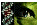Table 3 of Gilliland, Mol Vis 2001; 7:120-130.

Table 3. Prediction of the number of MLBs per unit volume

The Floderus equation was used to determine the numerical density (Nv) of the MLBs. The number of MLBs per unit area, the section thickness (0.5 mm), and the average diameter of an MLB (2.36 mm) led to a determination that there are 10 times as many MLBs in the cataract than in the normal lens.

 ``` Normal Cataract -------- -------- NV (MLBs/mm3) 382 3887 VMLB/mm3 0.000003 0.00003 ```

These calculations are based on:

NV = NA/(T + d - 2h)

where

NV = Number of profiles of a feature/unit test volume (i.e., numerical density)
NA = Number of profiles of a feature/unit test area (measured, MLBs/mm2)
T = Section thickness = 0.5 mm (measured)
d = Profile diameter = 2.39 mm (measured average)
h = Height of the cap = 1/3 T (calculated)

Height of the cap (the smallest observed profile in the section) serves as a correction for the small polar regions of a sphere, which become lost to the eye since they are grazed during sectioning and therefore are only present in part of one section. For further information on the Floderus equation, see Bozzola and Russell .

Gilliland, Mol Vis 2001; 7:120-130 <http://www.molvis.org/molvis/v7/a18/>